### SR #17: Relative LengthFlux Capacitor Charged!

Last time's Too Long Train illustration demonstrates that length is relative. Observers moving at different rates measure the length of an object differently. The faster something moves in your frame of reference, the more its length contracts along the direction of motion.

In previous weeks we saw that motion, speed, and simultaneity, are relative; now we see that length is also relative. Next week I'll talk about the relativity of time. Today I want to dig a little deeper into the length contraction part of Special Relativity.

It'll be a factor when we get to the spaceships!

We saw that a long train moving fast enough fits inside a tunnel.Behind Door #1: A train!

This length contraction isn't an illusion caused by how long it takes light to reach our eyes. As the closed tunnel doors prove, the train isfrom Al's point of view — definitely contracted along its direction of travel.

Remember: to the people on the train, nothing unusual is happening!

From their point of view, it's the passing world that seems contracted along its direction of travel. (The way space seems to contract plays an important role for speedy spaceships and time dilation. It's how a traveler — whose clock ticks normally — can go a long distance in what to them seems like a shorter time.)

We've seen how the lines of simultaneity rotate when we look at a moving frame. The faster that frame moves, the more the line of simultaneity rotates. If the speed is zero — if there's no motion — the line of simultaneity is horizontal, there's no rotation. At light speed, c, the fastest possible speed, the rotation is 45 degrees.

At the same time, the world line showing the motion of a person or object through a rest frame also rotates depending on the speed. World lines rotate from strictly vertical when speed is zero to 45 degrees at light speed.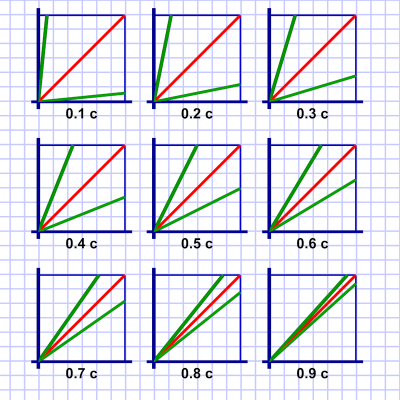Diagram 1. The angle of world and simultaneity lines at different fractions of c. The upper green line is the world line of someone passing by at some fraction of c. The lower green line is their line of simultaneity. The red line show light speed's 45-degree angle.

In both cases the angle varies from zero to 45 degrees — the angle our diagrams show for light's motion. That's no coincidence. As relative speed increases, both the world and simultaneity lines approach 45 degrees.

At light speed, they become parallel with light. The implication is that anything traveling at light speed is frozen in time; its clock never ticks. The only thing we know that does travel at light speed is light.

Photon clocks do not tick!

I'll talk about this more another time. Right now I want to get back to length contraction. Let's start by parking the train on the track so it's just sitting there: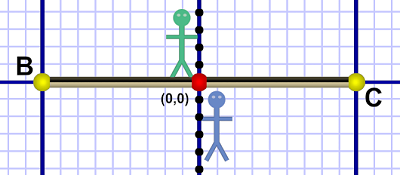Diagram 2. Train parked on tracks. No one is moving.

Em, as usual, is on the train; she's standing at the midway point. Al is standing on the ground just outside Em's window. They're both standing at the x=0 distance origin. (Nothing's moving, so we won't worry about time for the moment.)

Both Al and Em agree the train fits between points B & C. Al can mark those points with traffic cones, or lightning strikes (if he has a pair handy), or we could say B & C are the entrance and exit of a tunnel. They are points in Al's frame of reference, and they represent a distance in that frame.

Now that the train itself was used to mark its length, Em backs up and then drives past Al at a sedate speed of, let's say, 80 MPH.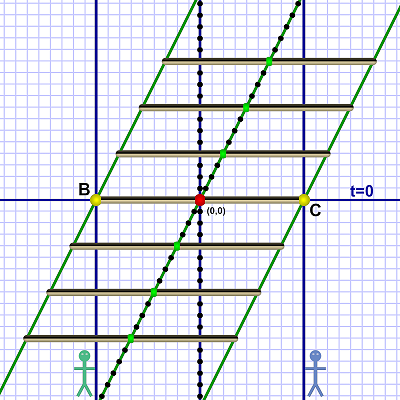Diagram 3. Em drives slow train past Al.

Since Em is moving so slowly (compared to light) she doesn't experience any Special Relativity effects. Neither does the train. As it passes Al at his t=0 line, he sees that it fits exactly between the markers.

More importantly, any tests either of them do will show they both map all points of the ground, track, and train, the same way. Their lines of simultaneity match (no rotation). Their clocks all tick in synch and all show the same time.

This time Em backs up a long ways, puts the hammer down, and returns to Al's position at relativistic speed. She passes him at 0.5 c (half the speed of light):Diagram 4. Em drives fast train past Al.

Now SR kicks in, and Em's line of simultaneity is rotated. Diagram 4 has three snapshots of the train from Em's perspective when: (1) the front of the train reaches the C event; (2) Em (sitting mid-train) reaches the origin point; (3) the rear of the train reaches the B event.

As discussed yesterday, Em and the train experience these as sequential events, whereas Al sees them happen simultaneously.

A crucial point here is that while the diagram shows the train at an angle, Al slices time horizontally. Any snapshot he makes only sees what's simultaneous in his frame of reference right now: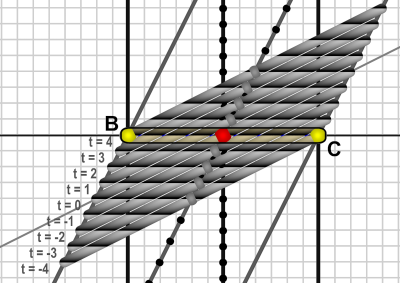Diagram 5. Al sees only what's between B & C.

Diagram 5 is a more filled-in version of diagram 4 — it shows nine snapshots of the train from (Em's) -4t to +4t.

Al's snapshot of the "train" between B & C includes parts of all nine plus all the in-between snapshots (an infinite number). The train Al sees has a smooth continuum of slices from different trains taken from a range of moments in Em's frame of reference.

It so happens that the geometry of those time slices requires the appearance of length contraction along the direction of travel. Just remember that there is no actual physical compression. It that the time slices of a moving object stack together to make it look that way!Time Continuum Train! Made of moments past, present, & future!

We're basically done for the day at this point.

There's a technical point I want to discuss if you care to keep reading…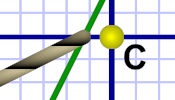Falling 86% short!

In diagram 4, you may have noticed that the front and rear of the train fell short of events C & B. Because of length contraction the train now fits between the markers with room to spare (at 0.5 c, the train is about 86% of its normal length).

The point I want to make is that it's not as simple just rotating lines on a drawing. The angle of the world line comes from the speed of the moving object given a scale where light moves at a 45-degree angle.

An object moving at light speed moves left or right one (space) unit for every vertical (time) unit. An object moving at half of c moves a half unit in space for every vertical unit. Obviously, if the speed is zero, there is no left or right movement.

The speed of the object determines its angle from vertical (zero) to 45 degrees (c). The simultaneity axis mirrors the angle off the horizontal axis; that gives us the angle of the line of simultaneity.Just rotating doesn't work

But if we just rotate the train (which is on the simultaneity axis), we get the wrong picture.

The ends of the train trace world lines that parallel Em's world line. (Naturally — Em and all parts of the train are in the same frame and move together.)

But in the diagram to the right, the ends of a merely rotated train fall way too short of the B & C markers. Al's view of such a train passing would be of a much shorter train than SR theory predicts.

So the geometry we need is a little more than just rotating the simultaneity axis. Obviously the train on that axis needs to be "longer" for the end points to sweep past the appropriate short-fall points.

If we accept the SR formula for length contraction, we know that at 0.5 c our gamma (γ) is 1.1547. The inverse of that (1/γ) is 0.866, which is where I got the "about 86%" just above.Lorentz shifting does

So if we know the ends of the train are 86% short of B & C, we can start from those points on Al's t=0 line. Then we draw lines that parallel  Em's world line (aka the train's midpoint world line).

We know the angle of Em's simultaneity axis; it's the mirror of the angle of her world line. Where the simultaneity axis meets the outer lines, that has to be the ends of the train on the diagram.

As you can see, it extends quite a ways beyond the B & C markers. There's an involved geometric relationship between the angle (which depends on the speed) and the end point lines. As speed increases, and both angles converge towards 45 degrees, the end point lines converge towards the center.

That makes the train appear shorter and shorter on the horizontal t=0 line. But the train's length on the diagram gets longer and longer! The front of the train is stretched further into Al's future while the back is stretched further into his past.

 (I didn't mention it in the Simultaneous Lightning Strikes example, but the strikes that hit the front and rear of the train did hit a train foreshortened by its motion. The bolts struck closer to each other than they would have had the train been standing still.)

 To re-emphasize: The train only appears foreshortened in our frame of reference (assuming we're standing on the ground with Al). The storm is part of that reference frame which includes the ground, the track, Al, and us. So the storm — and lightning bolts — see a foreshortened train.

 Just to point out that any actual train moving at c/2 pretty much instantly turns to plasma and causes an H-bomb type explosion.

 For those keeping score: 26.56 degrees off horizontal on our diagrams.